# Clustering & Types Of Clustering

By Learnbay Category Machine Learning Reading time 7-9 mins Published on Nov 17, 2020
##### Table of content

Clustering & Types Of Clustering is the process of finding similar groups in data, called a cluster. It groups data instances that are similar to each other in one cluster and data instances that are very different(far away) from each other into different clusters. A cluster is, therefore, a collection of objects which are “similar” between them and are “dissimilar” to the objects belonging to other clusters.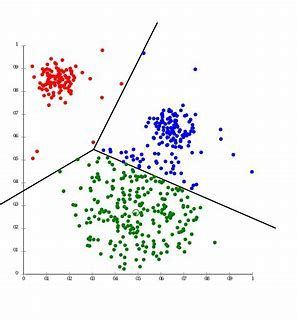The method of identifying similar groups of data in a dataset is called clustering. It is one of the most popular techniques in data science. Entities in each group and is comparatively more similar to entities of that group than those of the other groups. In this article, I will be taking you through the types of clustering, different clustering algorithms and a comparison between two of the most commonly used clustering methods.

Steps involved in Clustering analysis:

1. Formulate the problem – select variables to be used for clustering.

2. Decide the clustering procedure whether it will be Hierarchical or Non-Hierarchical.

3. Select the measure of similarity or dissimilarity.

4. Choose clustering algorithms.

5. Decide the number of clusters.

6. Interpret the cluster output(profile the clusters).

7. Validate the clusters.

## Types of clustering technique:-

Broadly speaking, clustering can be divided into two subgroups :

• Hard Clustering: In hard clustering, each data point either belongs to a cluster completely or not. For example, in the above example, each customer is put into one group out of the 10 groups.
• Soft Clustering: In soft clustering, instead of putting each data point into a separate cluster, a probability or likelihood of that data point to be in those clusters is assigned. For example, from the above scenario, each customer is assigned a probability to be in either of 10 clusters of the retail store.

Types of clustering are:

k-means clustering:

k-means clustering is a method of vector quantization, originally from signal processing, that is popular for cluster analysis in data mining. k-means clustering aims to partition n observations into k clusters in which each observation belongs to the cluster with the nearest mean, serving as a prototype of the cluster. This results in a partitioning of the data space into Voronoi cells. k-Means minimizes within-cluster variances (squared Euclidean distances), but not regular Euclidean distances, which would be the more difficult Weber problem: the mean optimizes squared errors, whereas only the geometric median minimizes Euclidean distances. Better Euclidean solutions can, for example, be found using k-medians and k-medoids.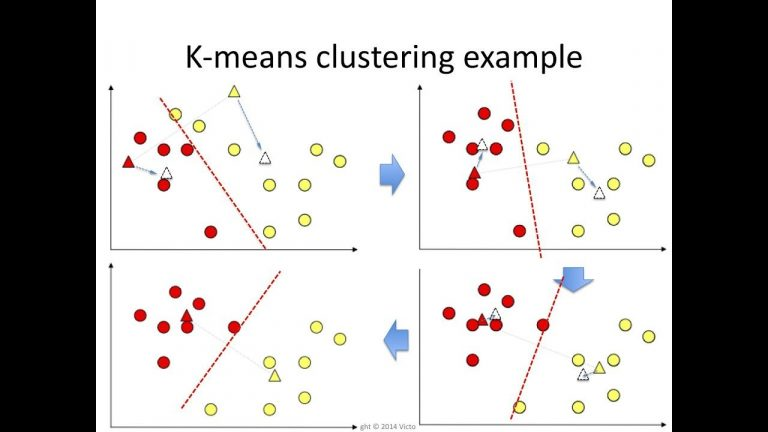K means is an iterative clustering algorithm that aims to find local maxima in each iteration. This algorithm works in these 5 steps :

1. Specify the desired number of clusters K : Let us choose k=2 for these 5 data points in 2-D space.
2. Randomly assign each data point to a cluster: Let’s assign three points in cluster 1 shown using red color and two points in cluster 2 shown using grey color.
3. Compute cluster centroids: The centroid of data points in the red cluster is shown using a red cross and those in a grey cluster using the grey cross.
4. Re-assign each point to the closest cluster centroid: Note that only the data point at the bottom is assigned to the red cluster even though its closer to the centroid of the grey cluster. Thus, we assign that data point into a grey cluster
5. Re-compute cluster centroids: Now, re-computing the centroids for both the clusters.
6. Repeat steps 4 and 5 until no improvements are possible: Similarly, we’ll repeat the 4th and 5th steps until we’ll reach global optima. When there will be no further switching of data points between two clusters for two successive repeats. It will mark the termination of the algorithm if not explicitly mentioned.
``````from pandas import DataFrame

* Data = {'x': [25,34,22,27,33,33,
31,22,35,34,67,54,57,43,50,57,59,
52,65,47,49,48,35,33,44,45,38,43,
51,46],

* 'y': [79,51,53,78,59,74,73,57,
69,75,51,32,40,47,53,36,35,58,59,
50,25,20,14,12,20,5,29,27,8,7] }

df = DataFrame(Data,columns=['x','y'])

print (df)

k-means for cluster=3

from pandas import DataFrame

import matplotlib.pyplot as plt

from sklearn.cluster import KMeans

Data = {'x': [25,34,22,27,33,33,
31,22,35,34,67,54,57,43,50,57,59,
52,65,47,49,48,35,33,44,45,38,
43,51,46],

'y': [79,51,53,78,59,74,73,57,69,
75,51,32,40,47,53,36,35,58,59,50,
25,20,14,12,20,5,29,27,8,7] }

df = DataFrame(Data,columns=['x','y'])

kmeans = KMeans(n_clusters=3).fit(df)

centroids = kmeans.cluster_centers_

print(centroids)

plt.scatter(df['x'], df['y'], c= kmeans.labels_.astype(float), s=50, alpha=0.5)

plt.scatter(centroids[:, 0], centroids[:, 1], c='red', s=50) Hierarchical Clustering:
``````

Hierarchical clustering, as the name suggests is an algorithm that builds the hierarchy of clusters. This algorithm starts with all the data points assigned to a cluster of their own. Then two nearest clusters are merged into the same cluster. In the end, this algorithm terminates when there is only a single cluster left.

The results of hierarchical clustering can be shown using the dendrogram. The dendrogram can be interpreted as: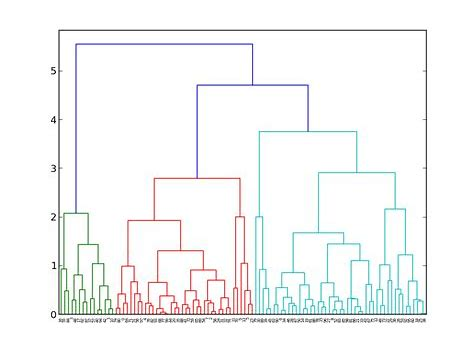Two important things that you should know about hierarchical clustering are:

• This algorithm has been implemented above using a bottom-up approach. It is also possible to follow the top-down approach starting with all data points assigned in the same cluster and recursively performing splits till each data point is assigned a separate cluster.
• The decision of merging two clusters is taken on the basis of closeness of these clusters. There are multiple metrics for deciding the closeness of two clusters :
• Euclidean distance: ||a-b||2 = √(Σ(ai-bi))
• Squared Euclidean distance: ||a-b||22 = Σ((ai-bi)2)
• Manhattan distance: ||a-b||1 = Σ|ai-bi|
• Maximum distance:||a-b||INFINITY = maxi|ai-bi|
• Mahalanobis distance: √((a-b)T S-1 (-b)) {where, s : covariance matrix}
``````import numpy as np

X = np.array([[5,3],

[10,15],

[15,12],

[24,10],

[30,30],

[85,70],

[71,80],

[60,78],

[70,55],

[80,91],])

import matplotlib.pyplot as plt

labels = range(1, 11)

plt.figure(figsize=(10, 7))

plt.scatter(X[:,0],X[:,1], label='True Position')

for label, x, y in zip(labels, X[:, 0], X[:, 1]):

plt.annotate(

label,

xy=(x, y), xytext=(-3, 3),

textcoords='offset points', ha='right', va='bottom')

plt.show()
``````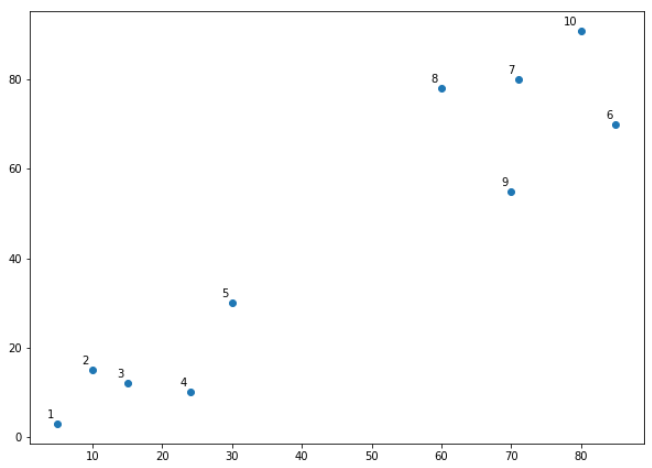from matplotlib import pyplot as plt

labelList = range(1, 11)

plt.figure(figsize=(10, 7))

orientation='top',

labels=labelList,

distance_sort='descending',

show_leaf_counts=True)

plt.show()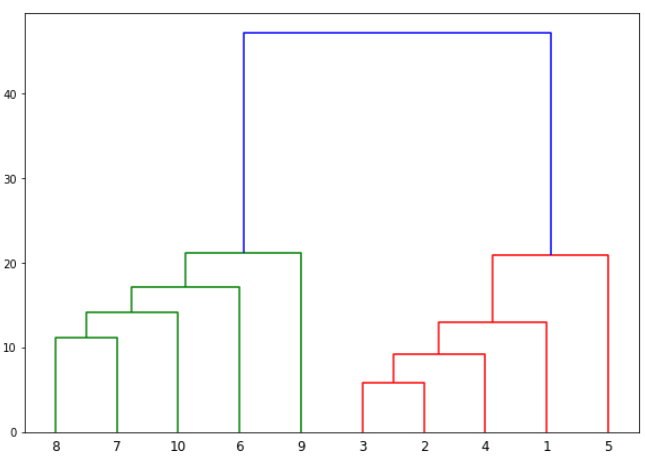Learnbay provides industry accredited data science courses in Bangalore. We understand the conjugation of technology in the field of Data science hence we offer significant courses like Machine Learning, Tensor Flow, IBM Watson, Google Cloud platform, Tableau, Hadoop, time series, R and Python. With authentic real-time industry projects. Students will be efficient by being certified by IBM. Around hundreds of students are placed in promising companies for data science roles. Choosing Learnbay you will reach the most aspiring job of present and future.

Learnbay data science course covers Data Science with Python, Artificial Intelligence with Python, Deep Learning using Tensor-Flow. These topics are covered and co-developed with IBM.